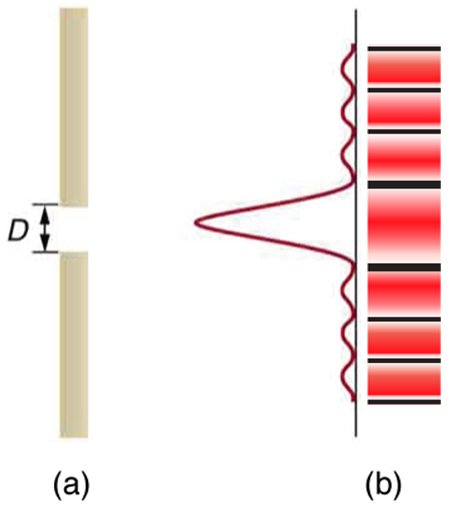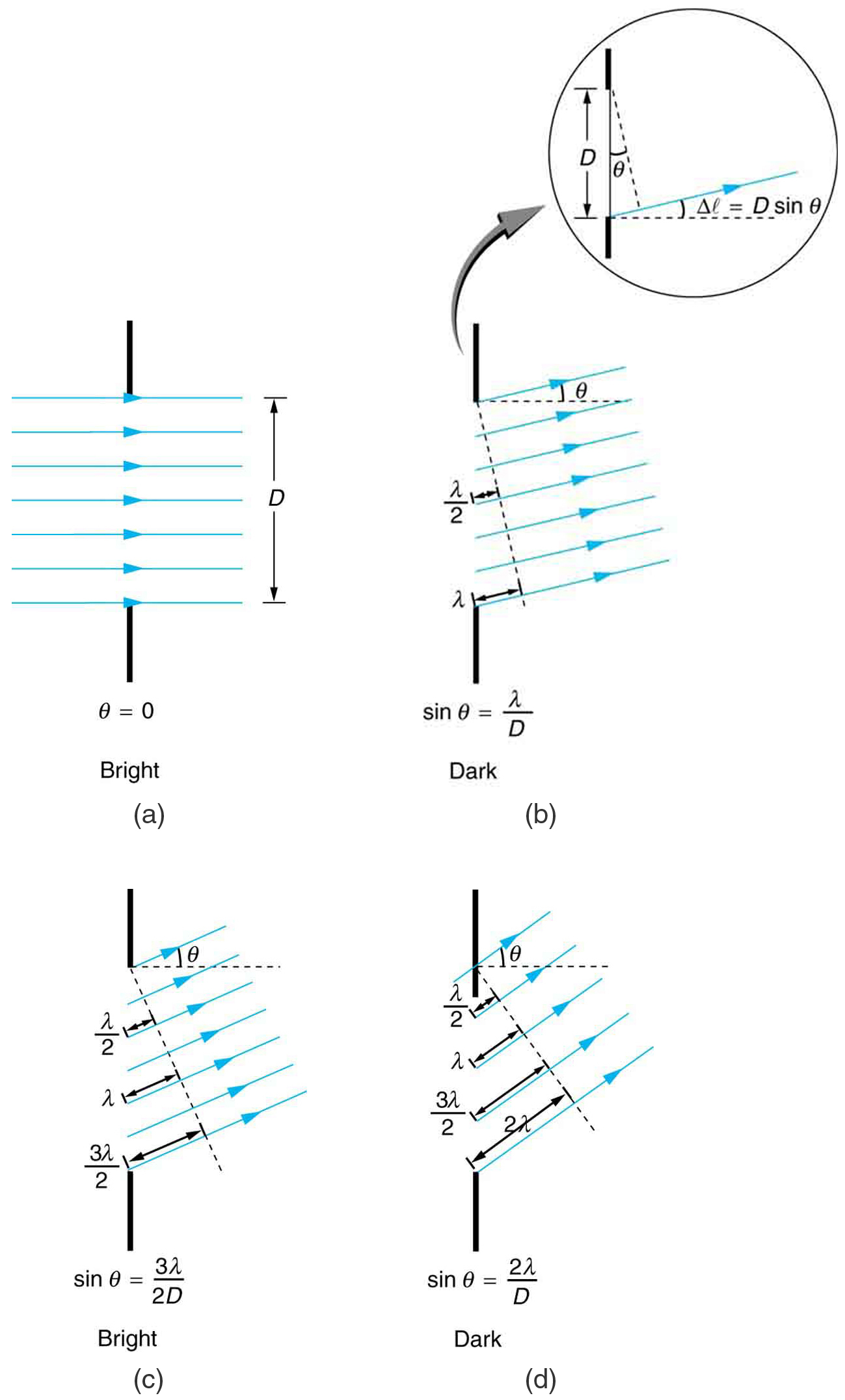# 27.5 Single slit diffraction

 Page 1 / 4
• Discuss the single slit diffraction pattern.

Light passing through a single slit forms a diffraction pattern somewhat different from those formed by double slits or diffraction gratings. [link] shows a single slit diffraction pattern. Note that the central maximum is larger than those on either side, and that the intensity decreases rapidly on either side. In contrast, a diffraction grating produces evenly spaced lines that dim slowly on either side of center.(a) Single slit diffraction pattern. Monochromatic light passing through a single slit has a central maximum and many smaller and dimmer maxima on either side. The central maximum is six times higher than shown. (b) The drawing shows the bright central maximum and dimmer and thinner maxima on either side.

The analysis of single slit diffraction is illustrated in [link] . Here we consider light coming from different parts of the same slit. According to Huygens’s principle, every part of the wavefront in the slit emits wavelets. These are like rays that start out in phase and head in all directions. (Each ray is perpendicular to the wavefront of a wavelet.) Assuming the screen is very far away compared with the size of the slit, rays heading toward a common destination are nearly parallel. When they travel straight ahead, as in [link] (a), they remain in phase, and a central maximum is obtained. However, when rays travel at an angle $\theta$ relative to the original direction of the beam, each travels a different distance to a common location, and they can arrive in or out of phase. In [link] (b), the ray from the bottom travels a distance of one wavelength $\lambda$ farther than the ray from the top. Thus a ray from the center travels a distance $\lambda /2$ farther than the one on the left, arrives out of phase, and interferes destructively. A ray from slightly above the center and one from slightly above the bottom will also cancel one another. In fact, each ray from the slit will have another to interfere destructively, and a minimum in intensity will occur at this angle. There will be another minimum at the same angle to the right of the incident direction of the light.Light passing through a single slit is diffracted in all directions and may interfere constructively or destructively, depending on the angle. The difference in path length for rays from either side of the slit is seen to be D sin θ size 12{D"sin"θ} {} .

At the larger angle shown in [link] (c), the path lengths differ by $3\lambda /2$ for rays from the top and bottom of the slit. One ray travels a distance $\lambda$ different from the ray from the bottom and arrives in phase, interfering constructively. Two rays, each from slightly above those two, will also add constructively. Most rays from the slit will have another to interfere with constructively, and a maximum in intensity will occur at this angle. However, all rays do not interfere constructively for this situation, and so the maximum is not as intense as the central maximum. Finally, in [link] (d), the angle shown is large enough to produce a second minimum. As seen in the figure, the difference in path length for rays from either side of the slit is $D\text{sin}\phantom{\rule{0.25em}{0ex}}\theta$ , and we see that a destructive minimum is obtained when this distance is an integral multiple of the wavelength.

Pls guys am having problem on these topics: latent heat of fusion, specific heat capacity and the sub topics under them.Pls who can help?
Thanks George,I appreciate.
hamidat
this will lead you rightly of the formula to use
Abolarin
Most especially it is the calculatory aspects that is giving me issue, but with these new strength that you guys have given me,I will put in my best to understand it again.
hamidat
you can bring up a question and let's see what we can do to it
Abolarin
the distance between two suasive crests of water wave traveling of 3.6ms1 is 0.45m calculate the frequency of the wave
v=f×lemda where the velocity is given and lends also given so simply u can calculate the frequency
Abdul
You are right my brother, make frequency the subject of formula and equate the values of velocity and lamda into the equation, that all.
hamidat
lExplain what happens to the energy carried by light that it is dimmed by passing it through two crossed polarizing filters.
When light is reflected at Brewster's angle from a smooth surface, it is 100% polarizedparallel to the surface. Part of the light will be refracted into the surface.
Ekram
What is specific heat capacity?
Specific heat capacity is the amount of heat required to raise the temperature of one (Kg) of a substance through one Kelvin
Paluutar
formula for measuring Joules
I don't understand, do you mean the S.I unit of work and energy?
hamidat
what are the effects of electric current
What limits the Magnification of an optical instrument?
Lithography is 2 micron
Venkateshwarlu
what is expression for energy possessed by water ripple
what is hydrolic press
An hydraulic press is a type of machine that is operated by different pressure of water on pistons.
hamidat
what is dimensional unite of mah
i want jamb related question on this asap🙏
What is Boyles law
it can simple defined as constant temperature
Boyles law states that the volume of a fixed amount of a gas is inversely proportional to the pressure acting on in provided that the temperature is constant.that is V=k(1/p) or V=k/p
what is motion
getting notifications for a dictionary word, smh
Anderson
what is escape velocity
the minimum thrust that an object must have in oder yo escape the gravitational pull
Joshua
what is a dimer
Mua
what is a atom
how to calculate tension
what are the laws of motion
MuaByByBy